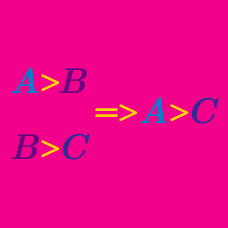Quantitative Finance

Induction - Problem Solving

Consider a statement $S(N): 1+3+5+\cdots+(2N-1)=7+N^2,$ then which of the following is true?

What is the remainder when ${105}^{165}-1$ is divided by 4?

Consider a sequence $\{a_n\}$ with $a_1=5$ and $a_2=13.$ If the sequence satisfies $a_{n+2}=5a_{n+1}-6a_n$ for all positive integers $n,$ what is $a_{50}?$

If $\displaystyle a_n=2^{2^n}+1$ for $n > 1,$ then what is the last digit of $a_{451}?$

Let $P(n)$ be a statement involving a positive integer $n.$ $P(n+2)$ is true if $P(n)$ or $P(n+1)$ is true. Then what is the sufficient condition for the statement $P(n)$ to be true for all positive integers?

×